CATEGORIES:

# STRAIGHT LINES IN SPACE

The Vector, Parametric, Canonical, and General Equations of a Straight Line

The position of a straight line in space is determined by a point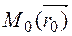on this line and a vector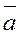parallel to the line. Let us write an

equation of such a line in space.

z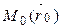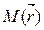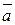0 y

x

To this end, we take an arbitrary point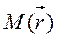on the line, join М0 and М to the origin, and find the coordinates of the radius-vectors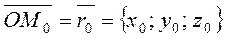,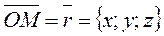.

It is seen from the figure, that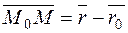.

If the point М belongs to the straight line, then the vectors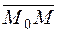and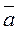are collinear.

Consequently, these vectors meet the collinearity condition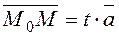,

where t is a parameter.

Let us write the collinearity condition in the form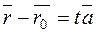; (*)

equation (*) is the vector equation of the given line.

Suppose given the coordinates of the point M0(x0,y0,z0) and the direction vector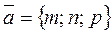. Let us write the left-hand side of equation (*) in the vector form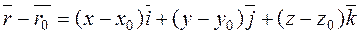the direction vector is.

Let us represent equation (*) in the form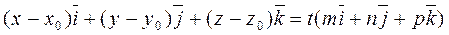.

Equating the respective coefficients of the unit vectors on the right- and left- sides, we obtain parametric equations of the straight line:or(27)

Eliminating the parameter t, we obtain the canonical equations of a straight line: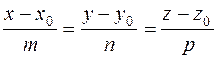. (28)

Example. Write the canonical equations of the straight line passing through the point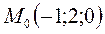parallel to the vector. We compose the canonical equation by formula (28):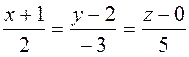.

Equating each fraction to a parameter t, we obtain the parametric equations of the line:The general equation of a straight line in space. Since a straight line in space is represented as the intersection of two planes, the general equation of a straight line in space has the form of a systemwhere the first and the second equations are the equations of the corresponding planes.

It is always possible to transform the general equation of a straight line into a canonical equation and vice versa.

Since the direction of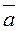is perpendicular to those of the vectors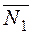and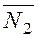, it follows that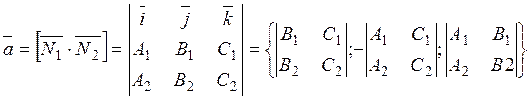,

i.e., the canonical equation is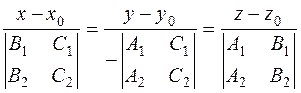.

The angle between straight lines in space. The parallelism and perpendicularity conditions for straight lines. Let us find the angle between intersecting right lines given by their canonical equations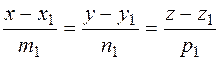;.

The angle between these two lines is equal to the angle between their direction vectors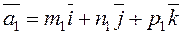;,

i.e.,.

The parallelism and perpendicularity conditions for right lines coincide with the collinearity and perpendicularity conditions of their direction vectors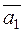and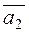.

If straight lines are perpendicular, then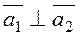, i.e.,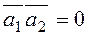, and the perpendicularity condition is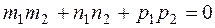.

If straight lines are parallel, then the vectoris collinear to, i.e., their coordinates are proportional, and the proportionality condition is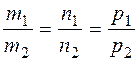.

Date: 2015-01-02; view: 2040

 <== previous page | next page ==> Since, the point belongs to the plane, its coordinates satisfy the general equation of the plane, and | The Intersection of a Plane and a straight Line
doclecture.net - lectures - 2014-2023 year. Copyright infringement or personal data (0.016 sec.)